# Epidemics:guineapigs:model

Society epidemics Guinea pigs epidemics Virus genetic evolution
Data | Model | Results

## Contents

### Model's assumptions

We have formulated a discrete stochastic model based on the probability of infection. To estimate this probability, we apply a simple generalized linear model (GLM), with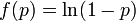$f(p) = \ln(1-p)$ as the link function. This is a standard approach that has been successfully adopted in various related contexts for modeling influenza. The model starts from day zero, when all non pre-infected animals are healthy. For each consecutive day, we calculate the probabilities that each individual, among those not yet infected, will be infected on that day, and then we randomly check whether any of the possible infections actually happens. The infection history is subsequently updated, and the algorithm moves to the next day.

We assume the four following conditions:

1. The probability that the ith individual will be infected on a particular day (provided that it had not been infected previously) is$p_i = 1 - \exp\left(-\gamma(T,H)\sum_j \alpha_{ij}\beta_j\right)$
(1)

where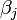$\beta_j$ is a measure of virus concentration in the respiratory tract of the jth individual (decimal logarithm of the number of PFU in one ml of its nasal wash). The summation goes over individuals placed on the same and on adjacent shelves. Spatial coefficients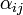$\alpha_{ij}$ are related to the probabilities that an aerosol drop, shed by the jth animal, will get to the ith guinea pig's cage. The coefficient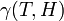$\gamma(T,H)$ reflects the dependence of the virus transmission rate on the air temperature (T) and relative humidity (H).

2. The course of influenza (virus concentration in nasal wash on the days subsequent to infection) depends only on the ambient temperature. Therefore, for each individual that became infected via air during the course of each particular experiment, the concentrations were assigned values reflecting the average case for that temperature. Table 1 shows the mean concentrations measured on particular days after infection, separately for each temperature. Functions comprising two linear pieces appear to be a reasonable guess, as shown in Figure 1.

 Day Concentration,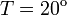$T = 20^\mathrm{o}$C Concentration,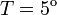$T = 5^\mathrm{o}$C Std. deviation square 1 2 3 4 5 6 7 8 9 1.52 4.52 5.63 6.89 6.06 3.26 1.88 — — 1.91 4.4 5.72 7.02 6.01 4.54 3.52 1.77 — 0.4 0.59 1.26 0.32 2.38 1.44 3.18 2.34 —

 Fig. 1: The two piecewise linear approximations of the infection course. For 20$^\mathrm{o}$C the best fit is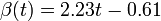$\beta(t) = 2.23 t - 0.61$ for the growing part and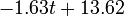$-1.63 t + 13.62$ for the falling; for 5$^\mathrm{o}$C it is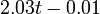$2.03 t - 0.01$ and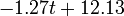$-1.27 t + 12.13$ respectively. We assume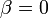$\beta = 0$ if the amount of virus is undetectable or zero

This may be interpreted as a two-phase infection course: first, the virus concentration grows exponentially (the scale is logarithmic), and subsequently (mostly as a result of the immune system activation) it exponentially falls. The transition between phase 1 and 2 is always sharp.

3. A detectable virus presence in the respiratory tract begins 2 days after the infection. Such an assumption is drawn from the fact that the virus was not detectable in nasal wash earlier than the third day of experiment. A lack of infections during the first day, when the virus concentrations in pre-infected individuals is supposed to be high, is of little probability. This is consistent with the major biological and clinical experience, which shows that the influenza proper begins rapidly after 2-3 days of a rather asymptomatic and non-infectious incubation period. Accordingly, we shifted the assumed average infection course such that$\beta = 0$ on the first day. The final concentration values are shown in Table 2.

4. The infection course in pre-infected individuals also consists of two linear (on a logarithmic scale) phases. Concentration values for the descending phase come from the measurement (We fitted a linear function for these values. However, they were originally of a nearly linear nature.). Due to the lack of the respective experimental data, for the ascending phase we assumed the slope to be the same as for animals infected via air. The virus concentration values for those individuals are also shown in Table 2.

 Day A, 20$^\mathrm{o}$C A, 5$^\mathrm{o}$C P, 20$^\mathrm{o}$C P, 5$^\mathrm{o}$C 1 2 3 4 5 6 7 8 9 10 0 1.62 3.85 6.08 7.08 5.45 3.82 2.18 0.55 0 0 2.02 4.05 6.08 7.06 5.79 4.52 3.25 1.98 0.72 5.07 7.3 6.7 5.42 4.14 2.87 1.6 0.32 0 0 4.97 7 8.54 7.32 6.1 4.87 3.64 2.42 1.2 0

### Propagation of infection

Assuming values for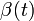$\beta(t)$,$\alpha_{ij}$, and$\gamma$, it is possible to compute the probability of each particular healthy individual becoming infected on the first day of the experiment (Equation 1). And from the probabilities determined for days 1 to$t$, we can calculate the value for day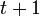$t + 1$ for each guinea pig in the following manner: for each sequence of days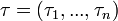$\tau = (\tau_1, ..., \tau_n)$, where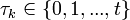$\tau_k \in \{0, 1, ..., t\}$, and$n = 4$ is the number of individuals to infect, let us take:

a) the probability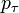$p_\tau$ that, for each$j$ from 1 to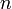$n$, the$j$th individual became infected on day$\tau_j$ (or has not yet been infected if$\tau_j = 0$) and
b) the sum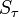$S_\tau$ that occurs in the exponent in Equation 1 if a) is the case.

The probability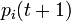$p_i(t + 1)$ that the$i$th individual will become infected on day$t + 1$ may then be considered as the expected value of a random variable which takes values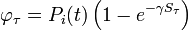$\varphi_\tau = P_i(t)\left(1 - e^{-\gamma S_\tau}\right)$

with probabilities$p_\tau$. Hence it equals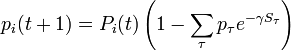$p_i(t + 1) = P_i(t)\left(1 - \sum_\tau p_\tau e^{-\gamma S_\tau}\right)$.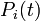$P_i(t)$ is the probability that the$i$th individual has not been infected previously, i.e., up to day$t$ (if it has, infection cannot happen anymore). Of course,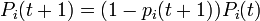$P_i(t + 1) = (1 - p_i(t + 1))P_i(t)$.

The above procedure establishes an iterative method for finding the infection probabilities on each consecutive day. The results are consistent with what was obtained from a number of Monte Carlo simulations, run with the same parameter values.

The coefficients$\alpha_{ij}$ indicate the fraction of the virus shed by the individual$j$ that contributes to infecting the individual$i$. We assume the following:

a)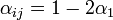$\alpha_{ij} = 1 - 2\alpha_1$ if the individual$j$ is located on the same shelf, in the adjacent column
b)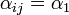$\alpha_{ij} = \alpha_1$ if it is located on an adjacent shelf, in the adjacent column
c)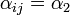$\alpha_{ij} = \alpha_2$ if it is located on an adjacent shelf, in the same column (so it is not pre-infected).

This is due to the fact that the virus particles shed by a particular animal from the pre-infected column will move mainly to the other cage located on the same shelf. However, a certain fraction of them,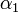$\alpha_1$, may diffuse to each of the two adjacent shelves (or out of the system if the source shelf is at the edge). In addition, a certain fraction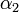$\alpha_2$ of the virus shed by individuals infected via air may also cross the shelf border and reach a neighboring animal in the same column. It seems reasonable to expect that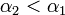$\alpha_2 < \alpha_1$.

We have already assumed that the virus concentration is always the same for all pre-infected individuals. The part of$S_\tau$ that comes from them is then equal to this concentration (multiplied by$1 - \alpha_1$ if the respective individual is located on the very top or bottom shelf).

### An infection space

The coefficients$\alpha_1$,$\alpha_2$, and$\gamma(T,H)$ must be determined experimentally. In order to do so, we introduce a 3-dimensional vector space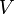$V$. The result of a particular experiment will be represented by a vector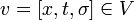$v = [x,t,\sigma] \in V$. The coordinates of this vector, in a certain base, have the following meaning:$x$ = the ratio of the individuals that were infected via air during the whole experiment to all the previously healthy individuals$t$ = the average time (number of days) elapsed before infection onset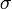$\sigma$ = standard deviation for the distribution of infection onset days.

We define a metric tensor on$V$ as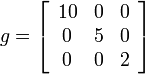$g = \left[\begin{array}{ccc} 10 & 0 & 0\\ 0 & 5 & 0\\ 0 & 0 & 2 \end{array}\right]$.

Thus, we are able to compute an abstract distance between two post-experimental states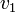$v_1$,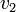$v_2$ by simply taking the square root of the scalar product of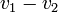$v_1 - v_2$ with itself: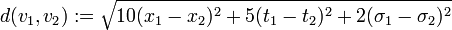$d(v_1, v_2) := \sqrt{10(x_1-x_2)^2 + 5(t_1-t_2)^2 + 2(\sigma_1-\sigma_2)^2}$.

This will allow comparison of the results of different experiments and simulations quantitatively. The diagonal terms of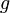$g$ have been chosen according to the range of variety of appropriate coordinates. We have set a large coefficient (equal to 10) for the total infection rate$x$ as it is a value between 0 and 1, and differs only slightly. On the other hand, the standard deviation of infection day,$\sigma$, is taken with a small coefficient (equal to 2) because it may differ considerably, even for simulations performed with similar parameter values. Note that this choice, although it seems reasonable, is arbitrary. Using another distance definition, it is possible to obtain quite different results.

Distances between points representing the experiments of Lowen et al. (2007) are collected in Table 3.

Tab. 3: Abstract distances between the experimental data points. The values in the right-upper corner correspond to temperature 20$^\mathrm{o}$C, and in the left-lower corner, to 5$^\mathrm{o}$C. We have taken the average V-coordinate values wherever there were two experiments performed under the same conditions
20% 35% 50% 65% 80%
20% 1.20 3.75 1.39 x
35% x 3.82 1.23 x
50% X 1.88 2.60 x
65% x 2.61 1.21 x
80% x 4.15 2.33 1.77

### Extraction of unknown parameters

Using the few experimental points in$V$, it is necessary to determine the unknown parameters$\alpha_1$,$\alpha_2$, and$\gamma(T,H)$. This is done by using the iterative algorithm described in section Propagation to generate a number of points corresponding to various reasonable parameter sets. Each of these sets will then be classified, separately for each temperature, as an approximation of parameter values for one particular humidity level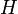$H$ corresponding to the available experimental data, namely, the one that is represented by the experimental point of least distance to the point considered. After that, a search is performed for the most relevant values of$\alpha_1$, and$\alpha_2$, that cover the maximal number of experimental cases for both temperatures and with the smallest possible distances. The last step involves determination of$\gamma$ values for each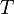$T$ and$H$ separately.

For the simulations here we set 17 irregularly spaced checkpoints for$\alpha_1$ and$\alpha_2$ from 0 to 0.5, and 62 checkpoints for$\gamma$ from 0.001 to 0.5. They are distributed more densely for lower parameter values.